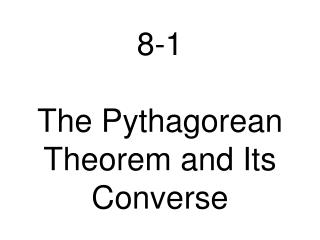DownloadDownload Presentation8-1 The Pythagorean Theorem and Its Converse

# 8-1 The Pythagorean Theorem and Its Converse

Download Presentation## 8-1 The Pythagorean Theorem and Its Converse

- - - - - - - - - - - - - - - - - - - - - - - - - - - E N D - - - - - - - - - - - - - - - - - - - - - - - - - - -
##### Presentation Transcript

1. 8-1The Pythagorean Theorem and Its Converse

2. Pythagorean Theorema2 + b2 = c2

3. Pythagorean Triples and Their Multiples3, 4, 55, 12, 13

4. ExampleIs a triangle with side lengths 9, 12 and 16 a right triangle?a2 + b2 = c2

5. ExampleIs a triangle with side lengths 9, 12 and 16 a right triangle?a2 + b2 = c2Which number goes in the c spot?

6. ExampleIs a triangle with side lengths 9, 12 and 16 a right triangle?a2 + b2 = c2Which number goes in the c spot?Why?

7. ExampleIs a triangle with side lengths 9, 12 and 16 a right triangle?a2 + b2 = c292 + 122 = 162

8. ExampleIs a triangle with side lengths 9, 12 and 16 a right triangle?a2 + b2 = c292 + 122 = 16281 + 144 = 256

9. ExampleIs a triangle with side lengths 9, 12 and 16 a right triangle?a2 + b2 = c292 + 122 = 16281 + 144 = 256225 = 256

10. ExampleIs a triangle with side lengths 9, 12 and 16 a right triangle?a2 + b2 = c292 + 122 = 16281 + 144 = 256225 = 256No

11. When you plug the 3 sides of a triangle into the Pythagorean theorem, if the c2 is larger then it is an obtuse triangle. If it is smaller then it is an acute triangle.

12. Examplea2 + b2 = c292 + 122 = 16281 + 144 = 256225 = 256Is this triangle Acute or Obtuse?

13. Turn to page 495.2, 3, 138-10, 19-30, 33, 36-385 Points: 100% Complete 4 Points: 80% Complete 3 Points: 60% Complete 2 Points: 40% Complete 1 Point: 20% Complete